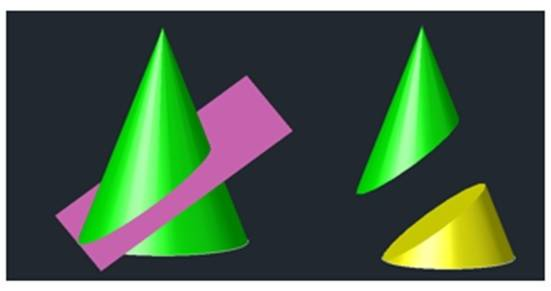# Problem about a cone and plane cutting it

• B
It has a straight cone and a plane that cuts it as shown in the image. What angle should the plane form with the base of the cone ?, so that the plane cuts the cone in 2 parts of equal volume.#### Attachments

berkeman
Mentor
Welcome to the PF. Is this question for schoolwork?

Hello, no, it's a cuirosidad. I try to resolve, however, I can not remember how.

berkeman
Mentor
Hello, no, it's a cuirosidad. I try to resolve, however, I can not remember how.
Fair enough. Do you remember how to use integration to calculate the volume between planes and other surfaces?

The problem is ill-posed. It has an infinitude of solutions as it's depending on the height of the plane above ground it cuts cone rather than the angle.

For example if my quick calculations are correct, for angle 0 degrees of plane parallel to ground a solution would be: ## h-\frac {h} { \sqrt 2} ##.

mfb
Mentor
Based on the image I guess the cutting plane should intersect the floor of the cone at the outer edge of the base.
The angle will still depend on the height/radius ratio but that dependence is trivial.

•berkeman
Based on the image I guess the cutting plane should intersect the floor of the cone at the outer edge of the base.
The angle will still depend on the height/radius ratio but that dependence is trivial.
I see..

Still it depends on the orientation of the plane then. Is the line of cut of the tilted plane with the z=0 plane the x=0 line, the y=x line? there are y=nx solutions...

For the simplest case, here's a reference: http://mathworld.wolfram.com/EllipticCone.html

Last edited:
jbriggs444
Homework Helper
Still it depends on the orientation of the plane then. Is the line of cut of the tilted plane with the z=0 plane the x=0 line, the y=x line? there are y=nx solutions...
One assumes the intersection of the tilted plane with the z=0 plane forms a line which is expected to be tangent to the base of the cone. And one assumes a right circular cone.

•berkeman and mfb
So. find the major and minor semi-axes a,b of the ellipse as functions of the angle of tilted plane and pluck them in ## a.b = r^2 /2 ##

Good luck with the calculations!

mfb
Mentor
OP asked about the volumes not about the area of the cut. The area of the cut will be larger than half the base.

OP asked about the volumes not about the area of the cut. The area of the cut will be larger than half the base.
Huh?

Volume of elliptic cone is half the volume of original cone. So, ##
π.a.b.h/3 = π.h.r^2 /6 =>
a.b = r^2 /2
##

Where did you see an area?

On second thought ##

a.b = r^2 /2 ## is not the right formula to be used...

The definition of an elliptic cone given in http://mathworld.wolfram.com/EllipticCone.html is not applicable in this case. What we are having here is a right circular cone cut by an oblique plane.

So in ##
π.a.b.h'/3 = π.h.r^2 /6 ## I would be using h' instead where h' is the height of the perpendicular drawn from the apex of the cone to the oblique cutting plane.

Yes, ##
π.a.b.h'/3 = π.h.r^2 /6 ## where h' is the height of the perpendicular drawn from the apex of the cone to the oblique cutting plane and h,r the height and radius of the original cone is the correct formula to be used!

mfb
Mentor
The upper volume is not an elliptic cone although it happens to have the same volume as one.
Yes, ##
π.a.b.h'/3 = π.h.r^2 /6 ## where h' is the height of the perpendicular drawn from the apex of the cone to the oblique cutting plane and h,r the height and radius of the original cone is the correct formula to be used!
That is better, and h'<h is the reason your previous approach didn't work.

Define ##L=\sqrt{h^2 + r^2/4}##, the distance between apex and the circumference of the intersection between cone and horizontal plane. Define ##\alpha## to be the angle between cone and horizontal surface and ##\gamma## to be the angle between cut plane and horizontal surface. Define ##\beta= \pi - 2 \alpha## (angle at the apex). Then ##h' = L \sin(\alpha - \gamma)##.
From the law of sines we get ##\frac{L}{\sin(\alpha+\gamma)} = \frac{a}{\sin(\beta)}##.
Now we just have to find b.

Can you move the plane?(Since I didn’t see any post above says that you can,t) if so,can you use the plane to cut the cone vertically in half?(This is quite ridiculous since some actual attempts have been mentioned above)